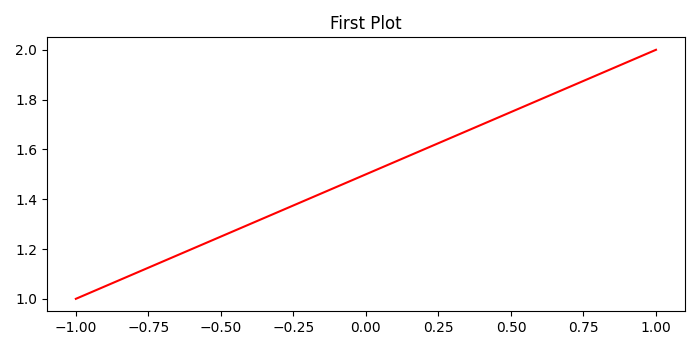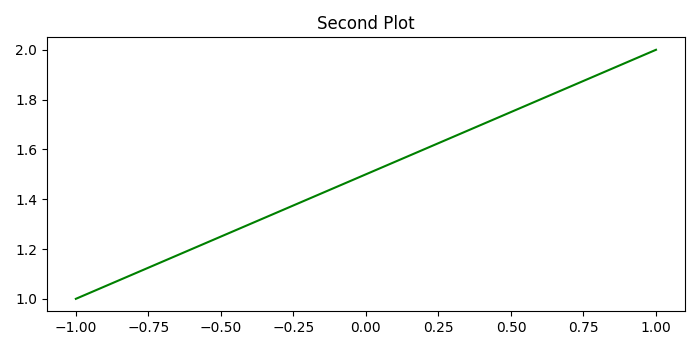# What is the difference between plt.close() and plt.clf() in Matplotlib?

• plt.figure() - Create a new figure or activate an existing figure.

• plt.figure().close() - Close a figure window.

• close() by itself closes the current figure

• close(h), where h is a Figure instance, closes that figure

• close(num) closes figure number num

• close(name), where name is a string, closes the figure with that label

• close('all') closes all the figure windows

• plt.figure().clear() - It is the same as clf.

• plt.cla() - Clear the current axes.

• plt.clf() - Clear the current figure.

## Example

import numpy as np
from matplotlib import pyplot as plt
plt.rcParams["figure.figsize"] = [7.00, 3.50]
plt.rcParams["figure.autolayout"] = True
x = np.linspace(-1, 1, 10)
y = np.linspace(1, 2, 10)

plt.plot(x, y, c='red')
plt.title("First Plot")
plt.show()
plt.clf()

plt.plot(x, y, c='green')
plt.title("Second Plot")
plt.show()
plt.close()

## Output

When we execute the code, it will show the First Plot −After that, since we have used plt.clf(), it will clear figure, but keep the window open to plot the Second Plot.After that, since we have used plt.close(), it will close the current window and release the memory.

Updated on: 08-May-2021

816 Views